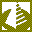Search
Software
Options for Equation Plotter - EqPlot
Equation Plotter - EqPlotGraph plotter program plots 2D graphs from complex equations. The application comprises algebraic, trigonometric, hyperbolic and transcendental functions. EqPlot can be used to verify the results of nonlinear regression analysis program.

Graphically Review Equations:
Equation graph plotter gives engineers and researchers the power to graphically review equations, by putting a large number of equations at their fingertips. The program is also indispensable for students and teachers.

Understandable and convenient interface:
A flexible work area lets you type in your equations directly. It is as simple as a regular text editor. Annotate, edit and repeat your graphings in the work area. You can also paste your equations into the editor panel.
Example of mathematical expression:
5.22 - (2 * x) + square(x) + power(x;3) + power(2.55;4) - logbaseN(4;6.25)
Save your work for later use into a text or graphic file. Comprehensive online help is easily accessed within the program.

Features:
--------
*Scientific graphings
*Unlimited expression length
*Parenthesis compatible
*Scientific notation
*More than 35 functions
*More than 40 constants
*User-friendly error messages
*Simple mode (medium size on desktop)
*Paste expressions into EqPlot
*Comprehensive documentation
*All the benefits that Windows bestows, such as multi-tasking and print formatting are available